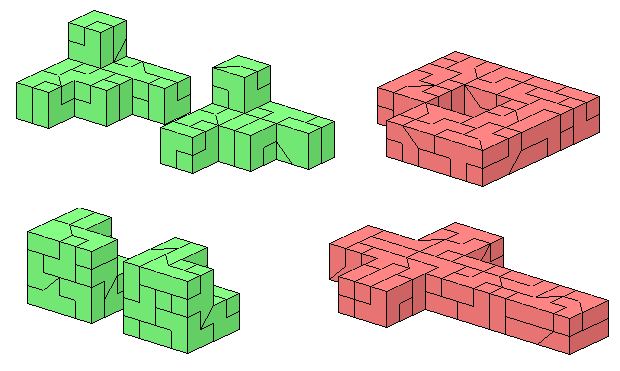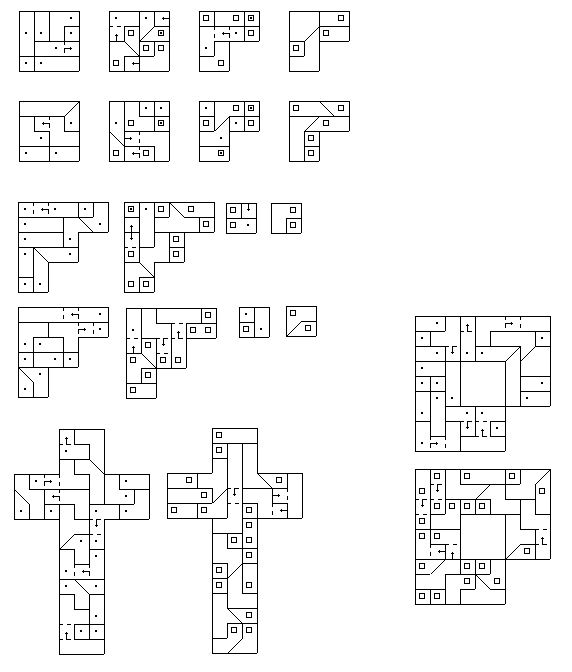# Models of Heptominoes and Heptacubes

With the 36 clipped tetracubes you can try to construct heptomino models three times as wide and two times as high with 7x3x3x2 = 126 = 3.5*36 unit cubes. As an example I built the latin cross and the hetomino with the hole. The 2x3x21 solid box is another example.
The total volume of the 32 extended tricubes is 32*3.5=112 = 2*7*8. Therefore two duplications of some or all? heptacubes may be possible. Two out of 1023 problems are solved here.Drawings showing the layers of the constructions.previous
next
back to index